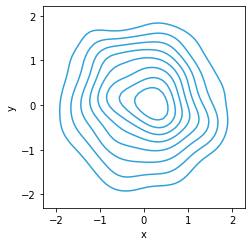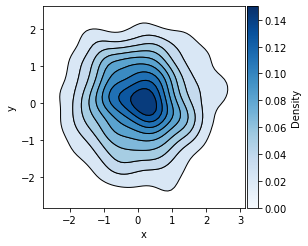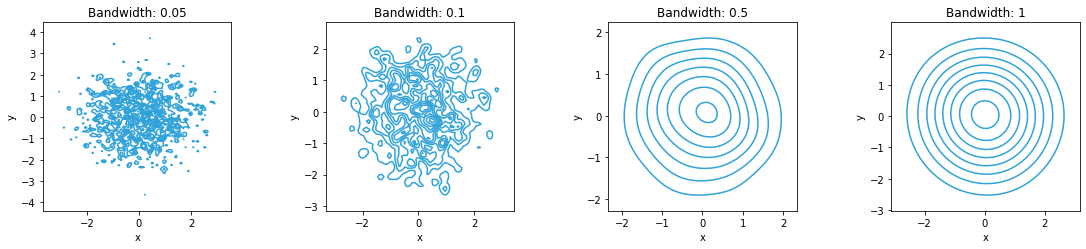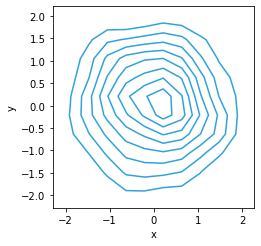# Bivariate#

Title
Bivariate Element
Dependencies
Matplotlib, SciPy
Backends
Matplotlib
Bokeh
```import numpy as np
import holoviews as hv

hv.extension('matplotlib')
````Bivariate` provides a convenient way to visualize a 2D distribution of values as a Kernel density estimate and therefore provides a 2D extension to the `Distribution` element. Kernel density estimation is a non-parametric way to estimate the probability density function of a random variable.

The KDE works by placing a Gaussian kernel at each sample with the supplied bandwidth, which are then summed to produce the density estimate. By default the bandwidth is determined using the Scott’s method, which usually produces good results, but it may be overridden by an explicit value.

To start with we will create a `Bivariate` with 1,000 normally distributed samples:

```normal = np.random.randn(1000, 2)
hv.Bivariate(normal)
```A `Bivariate` might be filled or not and we can define a `cmap` to control the coloring:

```hv.Bivariate(normal).opts(colorbar=True, cmap='Blues', filled=True)
```We can set explicit values for the `bandwidth` to see the effect. Since the densities will vary across the `NdLayout` we will enable axiswise normalization ensuring they are normalized separately:

```hv.NdLayout({bw: hv.Bivariate(normal).opts(axiswise=True, bandwidth=bw)
for bw in [0.05, 0.1, 0.5, 1]}, 'Bandwidth')
```Underlying the `Bivariate` element is the `bivariate_kde` operation, which computes the KDE for us automatically when we plot the element. We can also use this operation directly and print the output highlighting the fact that the operation simply returns an `Contours` or `Polygons` element. It also affords more control over the parameters letting us directly set not only the `bandwidth` and `cut` values but also a `x_range`, `y_range`, `bw_method` and the number of samples (`n_samples`) to approximate the KDE with:

```from holoviews.operation.stats import bivariate_kde
dist = hv.Bivariate(normal)
kde = bivariate_kde(dist, x_range=(-4, 4), y_range=(-4, 4), bw_method='silverman', n_samples=20)
kde
```For full documentation and the available style and plot options, use `hv.help(hv.Bivariate).`

This web page was generated from a Jupyter notebook and not all interactivity will work on this website. Right click to download and run locally for full Python-backed interactivity.